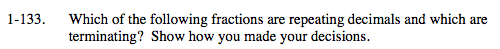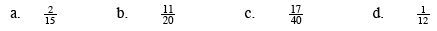### Home > MC2 > Chapter 1 > Lesson 1.3.2 > Problem1-133

1-133.Repeating Decimal: A decimal with numbers that follow a repeating pattern.
Terminating Decimal: A decimal that has a limited amount of number values.

Use standard long division or a calculator if necessary to examine the nature of the decimal values.

$\frac{2}{15} = 0.133$

Repeating

$\frac{11}{20} = 0.55$

Terminating

Based on these answers, see if you can determine whether parts (c) and (d) are repeating or terminating decimals.